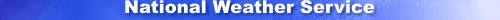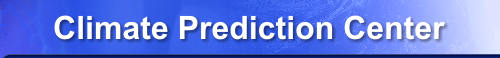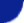Site Map News Organization SearchHOME > Monitoring_and_Data > Oceanic and Atmospheric Data > Reanalysis: Atmospheric Data > wgrib2-match_inv_add

Introduction !!ALPHA!!

Wgrib2's command line is a simple language that allows you to process selected fields using, for example, the -if option. The -if option is limited as it only works on parameters that are exposed by the -match_inv option. For example, you cannot check the diameter of the earth by using

wgrib2 IN.grb -if "code table 3.2=6 " -print "oh no code 3.2=6" -fi

because code table 3.2 is not in the match inventory (-match_inv).  The match
inventory has been expanding with time.  However, there will alway be new needs
that need an expanded match inventory.

You cannot add functions that depend on the grid point values (ex. -max, -min) or the locations of the grid points because these calculations are done after the match inventory is generated.

The current status is ALPHA and the syntax may be altered.

Usage

OPTION is an inv option with no parameters
note that you do not add a dash to the option
ARG1 is argument 1 to the option, if option has no argument, use a dummy argument
ARG2 is argument 2 to the option, if option does not need a second argument, use a dummy argument

As of v2.0.8, you are allowed to add upto 10 extra functions to the match inventory

Example

\$ wgrib2 small.grb2 -match_inv_add code_table_3.2 x x -match_inv
1:0:d=2009060500:HGT:200 mb:180 hour fcst:ENS=+19:HGT.ENS=+19:n=1:npts=4:var0_2_1_7_3_5:pdt=1: D=20090
605000000:start_FT=20090612120000:end_FT=20090612120000:scaling ref=1.22666e+06 dec_scale=-2 bin_scale
=2 nbits=12:code table 3.2=6 Earth assumed spherical with radius = 6,371,229.0 m:vt=2009061212:

\$ wgrib2 small.grb2 -match_inv_add code_table_3.2 x x -if "code table 3.2=6" -print "fount radius=6" -fi